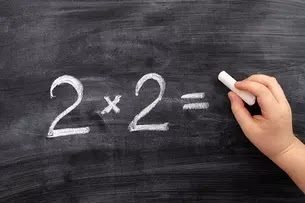# Memorizing Basic Multiplication with the Jarbel Method

Memorizing Basic Multiplication with  the Jarbel Method.  One problem of learning mathematics in elementary school is that it is difficult for students to operate numbers in certain material concepts. This can be seen from the facts that the author found in class.

After the teacher has finished explaining the concepts and formulations of the material to students by a certain method, they will easily answer the teacher's questions about the concept of the material that has been described. But once to the trial or practice section, after writing down the formula, the child will find it difficult to solve the given problem.

##Memorizing Basic Multiplication with  the Jarbel MethodWhat is Basic Multiplication

From there, the author knows the weak point of students in learning mathematics, namely the weak knowledge of basic multiplication,  Multiplication is not everything but everything in mathematics needs multiplication. Such is the conclusion when the author must be faced with the learning of mathematics at an advanced level.

This is because multiplication is one of the basic elements in the process of mathematical operations. This is a fact that many writers encounter in class. So memorizing the basic multiplication of 1-10 is an absolute requirement to be able to learn mathematics at a higher level. Multiplication learning has been taught since the second grade by instilling the concept that multiplication is a repeated summation.

## What is the Jarbel Method

To make it easier for students to memorize basic multiplication, the author uses the fingers of the hand to symbolize the numbers called the jarbel method . In addition to being easy and practical, this method of visualizing numbers with fingers can help students concentrate on the numbers being memorized. According to Sri Subarinah (2006: 31) the operation of multiplication by fingers is a method of counting that utilizes the fingers of the hand.

The use of fingers is used for multiplication of 6 to 9. This method is certainly very familiar. The author tried to share how to multiply the special number 9. Memorizing the multiplication of the number 9 can be done with the help of a 10-finger trick.

## How to Memorize Basic Multiplication with  the Jarbel Method

Following the reference, the position of the palm is aligned with the position of the back of the hand above. The right hand and all the fingers remaining on the right after one finger is folded are dozens. The left hand and all the fingers remaining on the left after one finger is folded are units.

Fold the finger as a multiplier number from right to left. So the right pinky represents the multiplier number How to Memorize Basic Multiplication by the Jarbel Method

As follows :

### Step Method Jarbel

1. The right ring finger represents the multiplier number two onwards until the left pinky which represents the multiplier number 10. Also read: The 4M Method in Mathematics of 2 x 9 Multiplication can be calculated by folding the right ring finger representing the multiplier number

2. After the right ring finger is folded, there will be 1 finger left in the right hemisphere and 8 fingers in the left hemisphere then 2 x 9 = 18. After the student is proficient in calculating multiplication operations with the fingers of his hand then the student is trained to memorize basic multiplication with a multiplication table of 1 to 10. Repetition is one of the important keys to memorizing the multiplication table.

So students need to prepare a complete multiplication table and to learn each student. Then every day students are asked to memorize a group of multiplication numbers in a loud voice of 2 to 5 repetitions. All for  numeracy literacy activities  of students in schools. Do it regularly every day until the student memorizes it outside the head and then continues with the next number.

So that the memorization of previous numbers is not lost, occasionally teachers give quizzes and give students rote bill cards. Multiplication is structured by involving parents, peers and teachers. This means that the first proof of memorization of the student must be signed by parents and then colleagues and finally the teacher as proof that the student has passed a certain multiplication.

## Conclusion

With the jarbel method,  which is an acronym for finger and multiplication table, fifth-grade students of SD Negeri 01 Supublor, Sragi District,  Pekalongan Regency, almost 75 percent have memorized basic multiplication of 1-10. This is very useful as a capital to be able to solve other mathematical problems. Such is the information about Memorizing Basic Multiplication by  the Jarbel Method.  Hope it is useful.